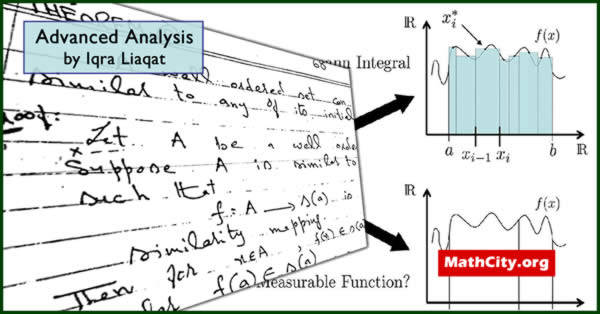# Advance Analysis by Ms. Iqra LiaqatThese notes are send by Ms. Iqra Liaqat. We are really very thankful to her for providing these notes and appreciates her effort to publish these notes on MathCity.org

 Name Advance Analysis Ms. Iqra Liaqat Unknown 222 pages PDF (see Software section for PDF Reader) 7.92 mB

In this section, the main heading of the definitions are given below.

• Rational numbers
• Proper subset
• Function
• One-one function, onto function
• Equivalence set
• Infinite set
• Denumerable set
• Countable set
• Uncountable set
• Choice functions
• Cardinal number
• Finite cardinal number
• Exponent of cardinals
• Schroeder Bernstein theorem
• Continum hypothesis
• Cantor's theorem
• Set theory
• Partially and totally ordered set
• Similar sets
• Well ordered set
• Ordinal numbers
• Axiom of choice
• Zorn's lemma
• Well ordered sets and ordinal numbers
• Principle of transfinite induction
• Limit elements
• Multiplication of ordinals
• Zorn's lemma
• Choice functions
• Set function
• Pre-measure
• Outer measure
• Monotone set function
• Sum of two outer measure
• Scalar multiplication of an outer measure
• Difference of two outer measure
• Lebesgue outer measure
• $\sigma$-Algebra
• Borel field
• Lebesgue measure
• Measurable function
• Characteristics functions
• Simple function
• Riemann integration
• Monotone convergence theorem
• Convergence in measure